# CPCTC

CPCTC

CPCTC is an acronym for "corresponding parts of congruent triangles are congruent'.

When two or more triangles are congruent then their corresponding sides and angles are congruent.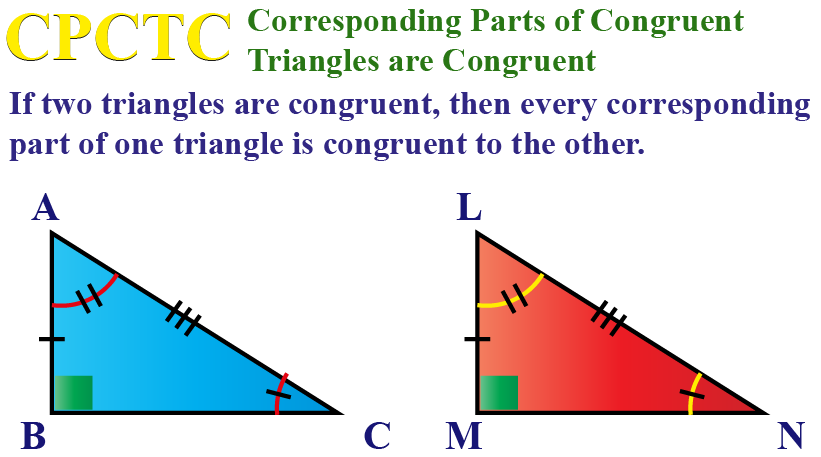## Lesson Plan

 1 What is CPCTC? 2 Thinking Out of the Box! 3 Important Notes on CPCTC 4 Solved Examples on CPCTC 5 Interactive Questions on CPCTC

## What is CPCTC?

The CPCTC theorem states that when two triangles are congruent, then their corresponding parts are equal.

When $$\triangle \text{ABC}\cong\triangle{DEF}$$, the three pairs of sides and three pairs of angles of $$\triangle \text{ABC}$$ = the three pairs of corresponding sides and three pairs of corresponding angles of $$\triangle \text{DEF}$$.

### Congruent Triangles

Two triangles are said to be congruent if they have exactly the same size and the same shape.

The equal sides and angles may not be in the same position.

### Corresponding Parts

Corresponding sides mean the three sides in one triangle are in the same position or spot as in the other triangle.

Corresponding angles mean the three angles in one triangle are in the same position or spot as in the other triangle.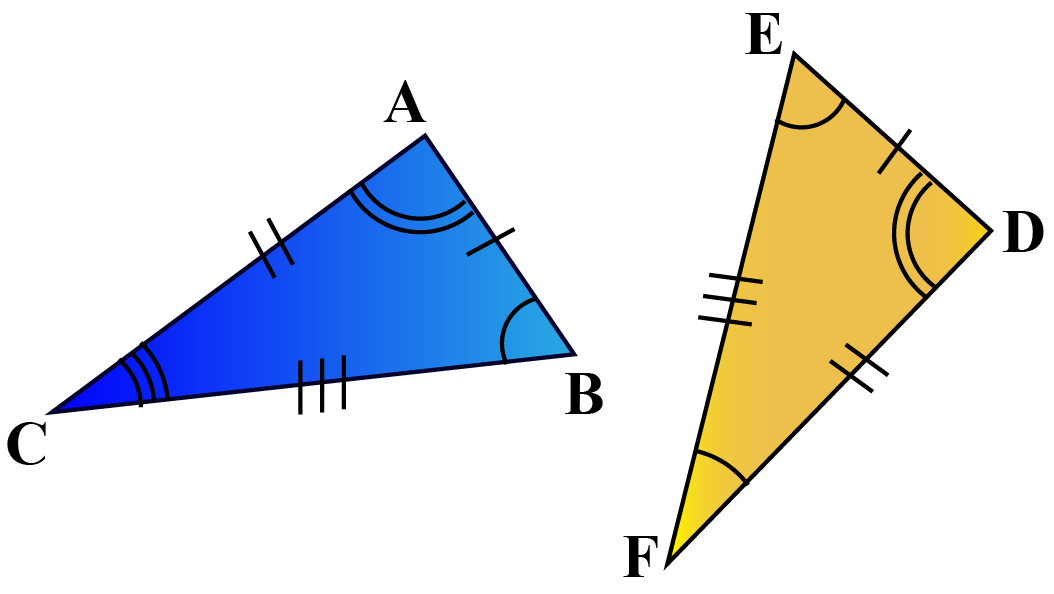### Explanation

In these two triangles ABC and DEF, let us identify the 6 parts: i.e. the three corresponding sides and the three corresponding angles.

AB corresponds to DE, BC corresponds to EF, CD corresponds to FD.

$$\angle$$ A corresponds to$$\angle$$D,  $$\angle$$B corresponds to$$\angle$$E, $$\angle$$C corresponds to$$\angle$$F.

And if $$\triangle\text{ABC} \cong \triangle\text{DEF}$$, then as per the cpctc theorem, the corresponding sides and angles are equal.

i.e. AB = DE, BC = EF,CD = FD

$$\angle$$ A = $$\angle$$D,  $$\angle$$B = $$\angle$$E, $$\angle$$C = $$\angle$$F.

## Explain CPCTC Triangle Congruence

Example

CPCTC states that if two triangles are congruent by any method, then all ot the corresponding sides and angles are equal.

Consider the two triangles $$\triangle1$$ and $$\triangle2$$ in each of the cases.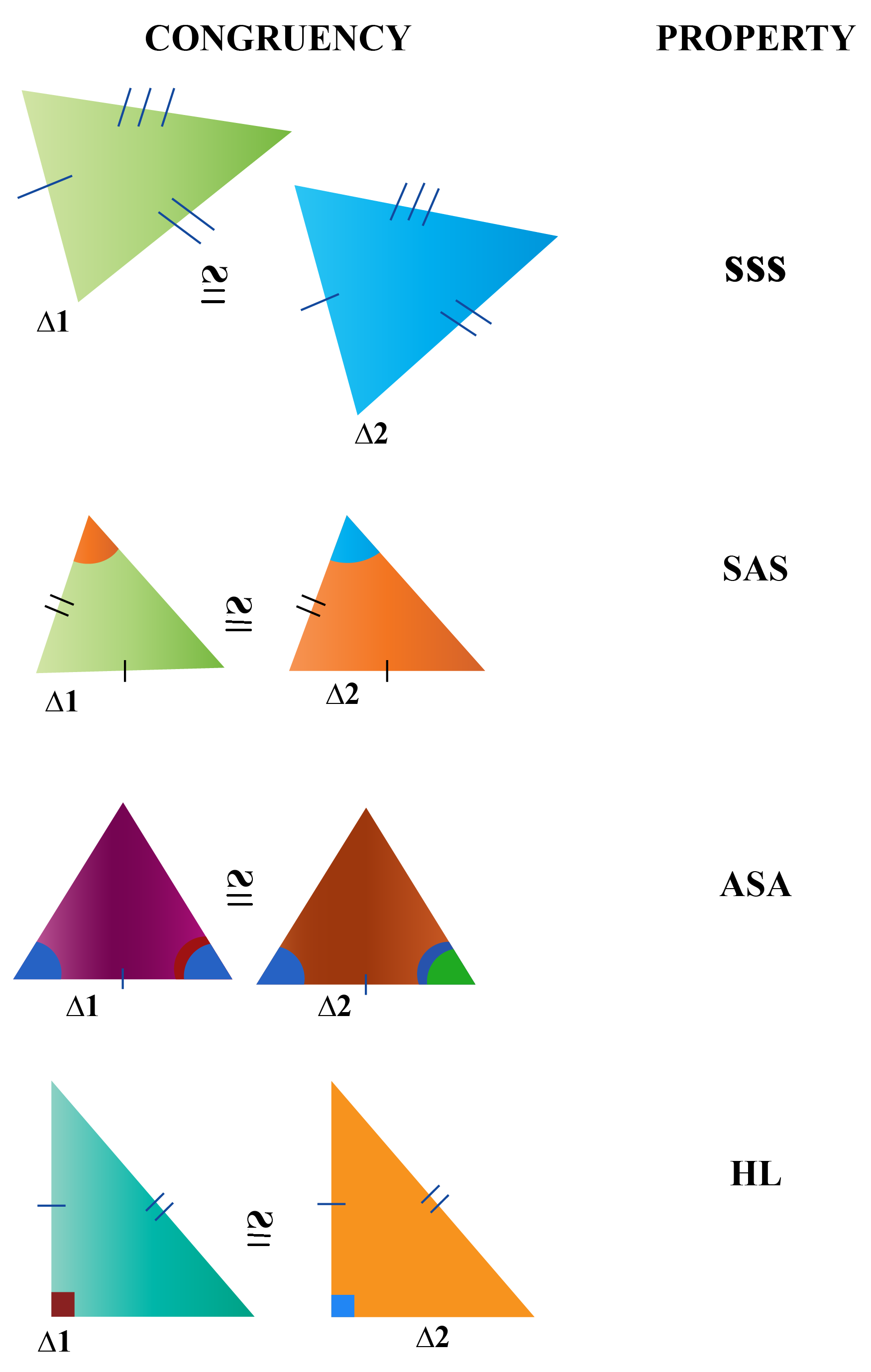### Explanation using CPCTC Worksheet

 Criterion Explanation CPCTC SSS All the 3 corresponding sides are equal. All the corresponding angles are also equal. SAS 2 corresponding sides and the included angle are equal. The other corresponding sides and the other 2 corresponding angles are also equal. ASA 2 corresponding angles and the included sides are equal. The other corresponding angles and the other 2 corresponding sides are also equal. RHS / HL The hypotenuse and one leg of one triangle are equal to the corresponding hypotenuse and a leg of the other. The other corresponding legs and the other two corresponding angles are equal.Think Tank
1. A related theorem is CPCFC, which is the acronym of congruent parts of congruent figures are congruent which applies to any polygon or polyhedron proven congruent.
2. Using CPCTC we prove many more theorems on parallelogram. A few are "Both pairs of the opposite sides of a parallelogram are congruent". "Both pairs of opposite angles are congruent." "Is Parallelogram, a rhombus?"

## How to Geometrically Prove CPCTC?

Consider triangles ABC and CDE. In your CPCTC worksheets, you can have the two-column proof done.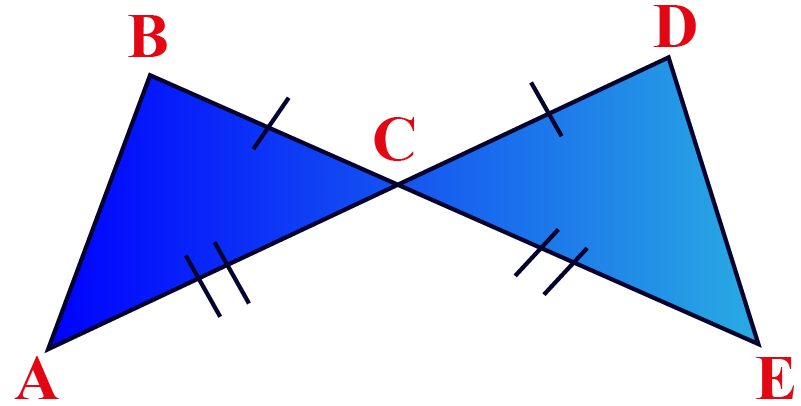### Proof

 Statement Reasons BC = CD Given AC = CD Given $$\angle ACB = \angle EDC$$ Vertically Opposite Angles are equal. $$\triangle\text{ABC} \cong \triangle\text{DEF}$$ By SAS (side-angle-side)criterion AB = DE, $$\angle$$ABC = $$\angle$$EDC and $$\angle$$BAC = $$\angle$$DEC CPCTC

### CPCTC Parallelogram

Example: Theorem: The diagonals of a parallelogram bisect each other.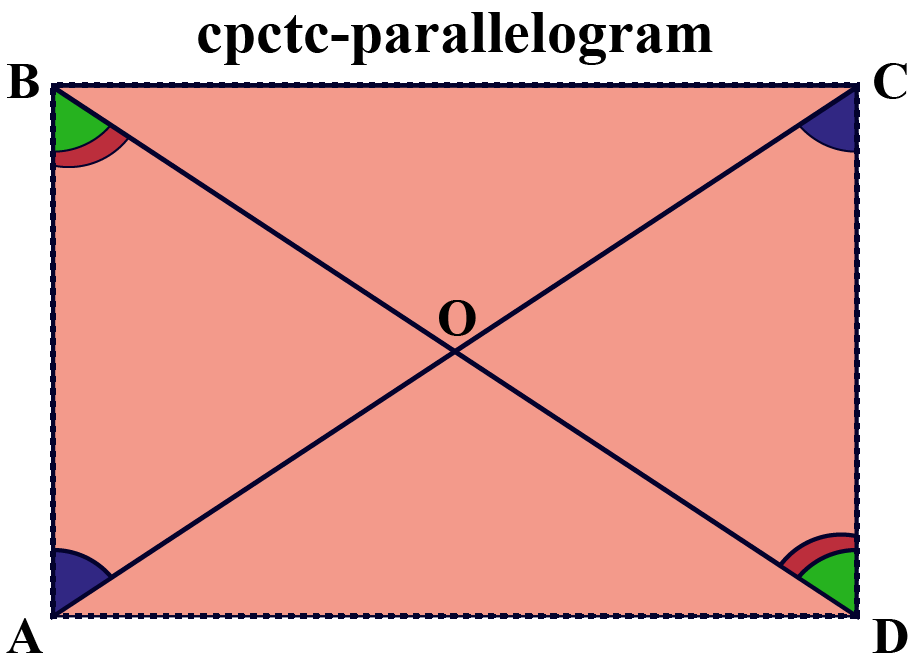### Explanation using CPCTC Worksheet

Statement Reason
AD = CB $$\because$$ opposite sides of parallelogram are equal
$$\angle$$DCA = $$\angle$$ CAB $$\because$$Alternate interior angles are equal.
$$\angle$$ABC = $$\angle$$ ADC $$\because$$Alternate interior angles are equal.
$$\triangle AOD \cong \triangle COB$$ By ASA Criterion
$$\therefore$$AO = CO , DO = BO By CPCTC

## Solved Examples

 Example 1

Find the length of LM.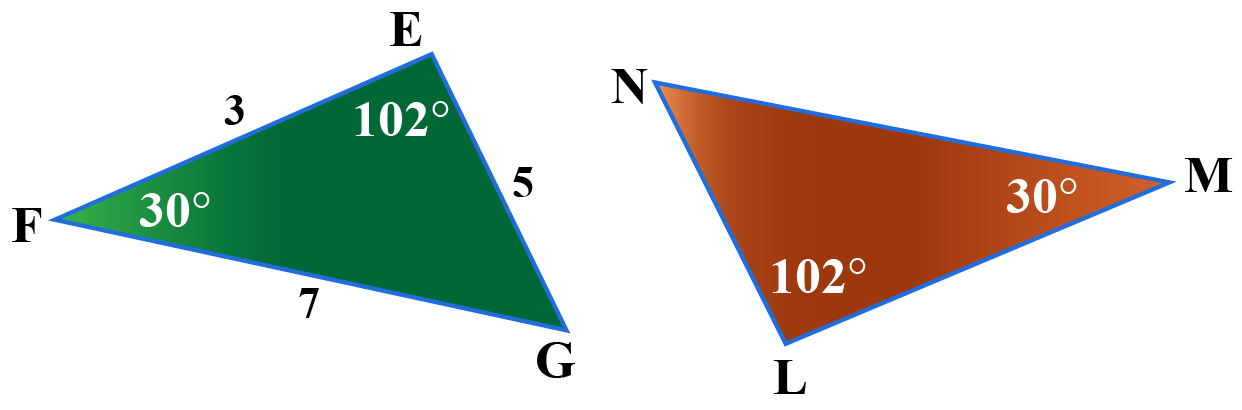Solution

Let us first find the missing angles.

In $$\triangle$$ EFG,

\begin{align}\angle EGF + \angle GFE + \angle FEG &= 180^{\circ}\\\angle EGF + 30^{\circ}+ 102^{\circ} &= 180^{\circ}\\\angle EGF &= 180^{\circ} - 132^{\circ}\\ &= 48^{\circ}\end{align}

In $$\triangle$$ LMN,

\begin{align}\angle LMN + \angle MNL+ \angle NLM &= 180 ^{\circ}\\\angle LMN + 48^{\circ}+ 102^{\circ} &= 180^{\circ}\\\angle LMN &= 180^{\circ} - 132^{\circ}\\ &= 30^{\circ}\end{align}

 Statement Reasons $$\angle$$ FEG= $$\angle$$ MLN Given $$\angle$$ EGF = $$\angle$$ MNL By Triangle sum property $$\angle$$ GFE = $$\angle$$ LMN By Triangle sum property $$\triangle$$ EFG = $$\triangle$$ LMN AAA criterion LM = EF = 3 CPCTC
 $$\therefore$$ LM = 3 units
 Example 2

Given: PR = RS. Find y.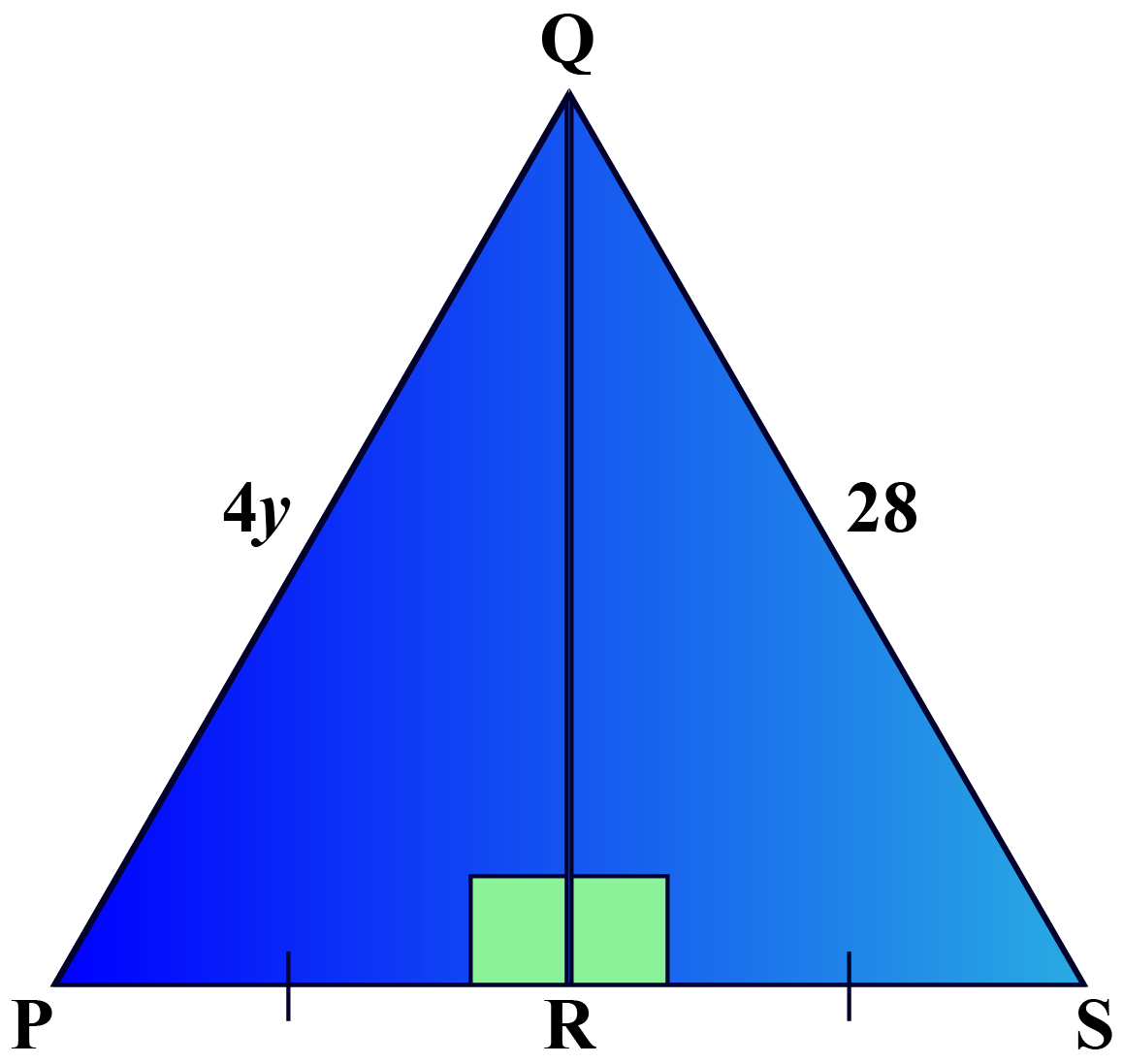Solution

 Statement Reason PR = RS Given QR = QR Reflexive property PQR = SPR HL criterion PQ = QS By cpctc

\begin{align} 4y &= 28\\y &= 28 \div4\\ &= 7 \end{align}

 $$\therefore$$y = 7 units.
 Example 3

Find angle BAC and angle DEC and b if the triangles ABC and CDE are congruent.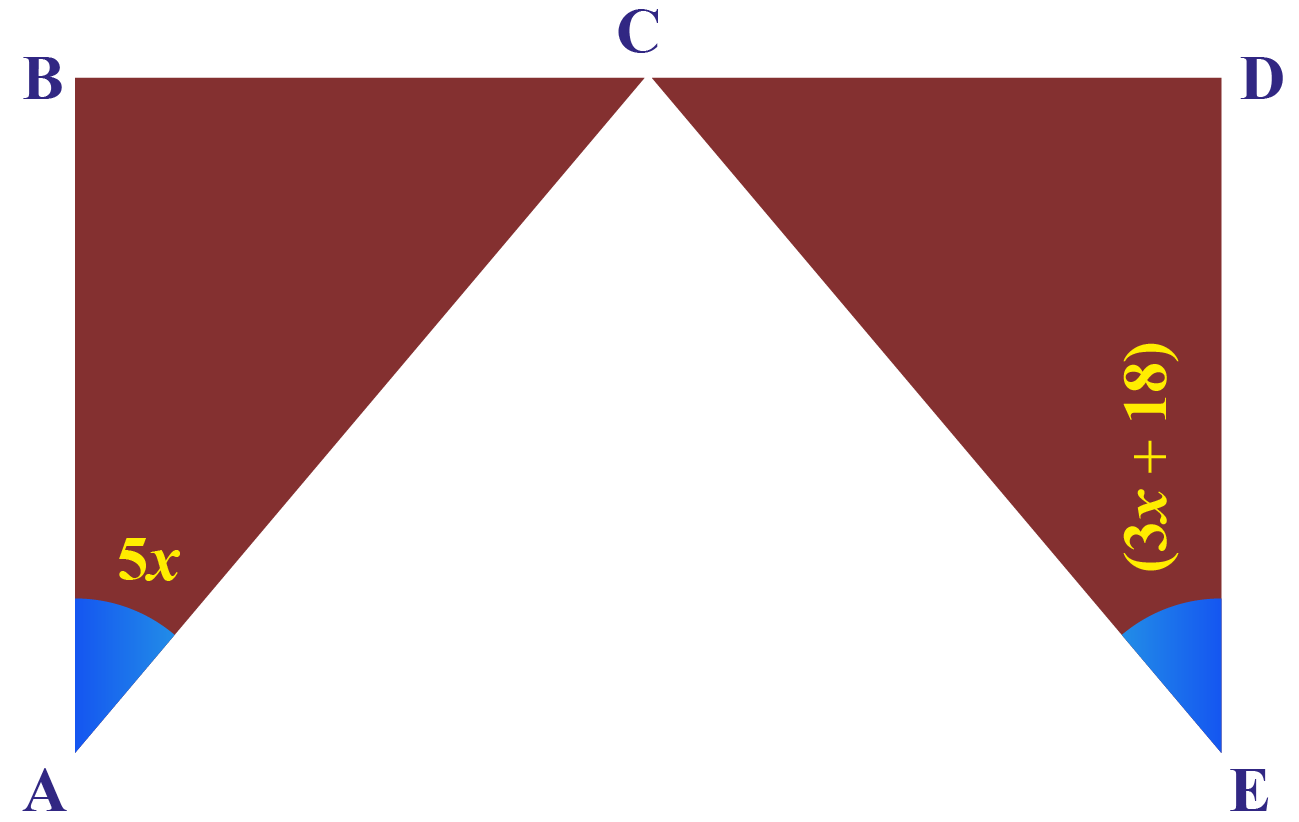Solution

Triangle BAC and triangle DEC are congruent.

By cpctc, we have $$\angle$$ BAC = $$\angle$$ DEC

\begin{align} \therefore 5x = 3x +18\\2x =18\\x= 9 ^{\circ}\end{align}
Substitute 9 in any of the angles.
\begin{align}5x = 5\times 9= 45 ^{\circ}\end{align}

 $$\therefore$$  $$\angle$$BAC = $$\angle$$ DEC= 45 $$^{\circ}$$

## Interactive Questions

Here are a few activities for you to practice. Select/Type your answer and click the "Check Answer" button to see the result.

## Let's Summarize

The mini-lesson targeted the fascinating concept of CPCTC. The math journey around CPCTC starts with what a student already knows, and goes on to creatively crafting a fresh concept in the young minds. Done in a way that not only it is relatable and easy to grasp, but also will stay with them forever. Here lies the magic with Cuemath.

## About Cuemath

At Cuemath, our team of math experts is dedicated to making learning fun for our favorite readers, the students!

Through an interactive and engaging learning-teaching-learning approach, the teachers explore all angles of a topic.

Be it worksheets, online classes, doubt sessions, or any other form of relation, it’s the logical thinking and smart learning approach that we, at Cuemath, believe in.

## 1. Is CPCTC a theorem?

Yes. CPCTC is a theorem that says corresponding parts of congruent triangles are congruent.

## 2. What is CPCTC for similar triangles?

Cpctc for similar triangles is not true. Corresponding angles of the two similar triangles are equal, whereas, corresponding sides of the triangles are not equal, but proportional.

## 3. How do you prove CPCTC?

After showing the proposed triangles are congruent, we can immediately say that the corresponding parts of congruent triangles are congruent.

More Important Topics
Numbers
Algebra
Geometry
Measurement
Money
Data
Trigonometry
Calculus